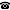# 2019 seminar talk: A Sacks amoeba forcing preserving distributivity of $P(\omega)/fin$

Talk held by Wolfgang Wohofsky (KGRC) at the KGRC seminar on 2019-05-09.

### Abstract

In my talk, I would like to present joint work with Otmar Spinas, in which we show that it is consistent that $\mathfrak{h}$ (the distributivity number of $P(\omega)/fin$, in other words, the least number of maximal almost disjoint families without a common refinement) is strictly smaller than $\mathrm{add}(s_0)$ (i.e., the least number of Marczewski null sets whose union is not Marczewski null, where a set is Marczewski null if each perfect set has a perfect subset disjoint from it). More explicitly, we show that this relation between $\mathfrak{h}$ and $\mathrm{add}(s_0)$ holds in the model obtained by a countable support iteration of length $\omega_2$ of a specific kind of Sacks amoeba forcing which happens to have the pure decision and the Laver property, and therefore does not add Cohen reals. The model actually satisfies $\mathfrak{h} = \mathrm{cov}(\mathcal{M}) < \mathfrak{b} = \mathfrak{s} = \mathrm{add}(s_0)$. (If time permits, I would also like to discuss why it seems easy to slightly modify the construction in such a way that the resulting model additionally satisfies the Borel Conjecture, but unclear how to modify it to make the Borel Conjecture fail.)

A video recording of this talk is available on Youtube.

Kurt Gödel Research Center for Mathematical Logic. Währinger Straße 25, 1090 Wien, Austria.+43-1-4277-50501. Last updated: 2010-12-16, 04:37.